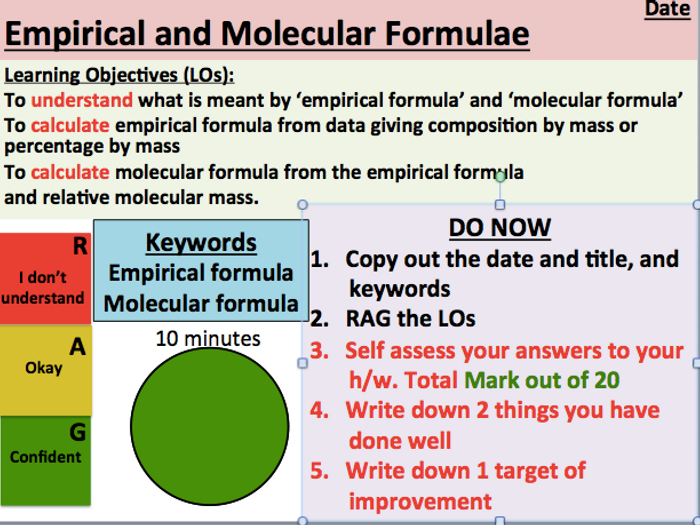Whole lesson including starter, main work tasks and answers on emprical and molecular formulae

Lesson begins with student self assessment on homework questions on moles and ideal gas equation (Search for KS5 ideal gas equation lesson)

By the end of the lesson students should be able to:

Understand what is meant by ‘empirical formula’ and ‘molecular formula’
Calculate empirical formula from data giving composition by mass or percentage by mass
Calculate molecular formula from the empirical formula and relative molecular mass.

\$8.37
Save for later

Info

Created: Nov 10, 2018

Updated: Mar 9, 2019

Whole lesson

pptx, 1 MB

Emprical-and-molecular-Formulae-

Lesson starter

docx, 84 KB

Homework-on-moles-and-ideal-gas-equation

pdf, 678 KB